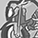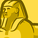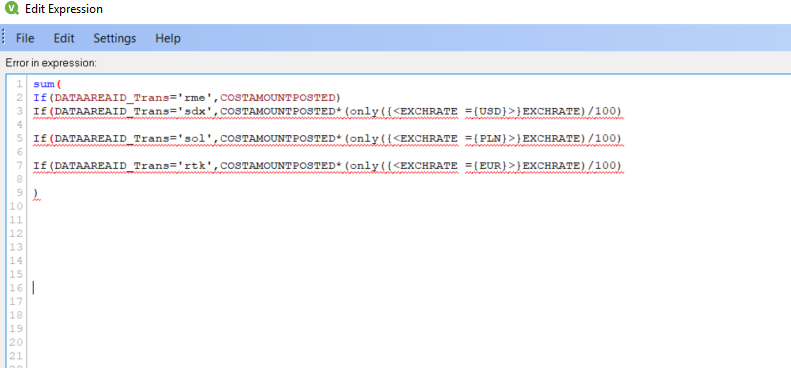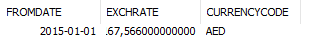# QlikView App Dev

Discussion Board for collaboration related to QlikView App Development.

Announcements
Our next Qlik Insider session will cover new key capabilities. Join us August 11th REGISTER TODAY
cancel
Showing results for
Did you mean:Contributor III

## Nested if statement

Hi,

I am trying to make and if statement in a chart expression with the following issues:

sum(

If(DATAAREAID_Trans='rme',COSTAMOUNTPOSTED)

If(DATAAREAID_Trans='sdx',COSTAMOUNTPOSTED*(EXCHRATE/100) where EXCHRATE = 'USD'

If(DATAAREAID_Trans='sol',COSTAMOUNTPOSTED*(EXCHRATE/100) where EXCHRATE = 'PLN'

If(DATAAREAID_Trans='rtk',COSTAMOUNTPOSTED*(EXCHRATE/100) where EXCHRATE = 'EUR'

)

Can anybody help me with this?

Kind regards Nicolai

1 Solution

Accepted SolutionsCreator III

Try this new Expression:

sum({<DATAAREAID_Trans={rme}>}COSTAMOUNTPOSTED)+

sum({<DATAAREAID_Trans={sdx}>}COSTAMOUNTPOSTED)*alt(max({<CURRENCYCODE ={USD}>}EXCHRATE /100),0)+

sum({<DATAAREAID_Trans={sol}>}COSTAMOUNTPOSTED)*alt(max({<CURRENCYCODE ={PLN}>}EXCHRATE /100),0)+

sum({<DATAAREAID_Trans={rtk}>}COSTAMOUNTPOSTED)*alt(max({<CURRENCYCODE ={EUR}>}EXCHRATE /100),0)+

sum({<DATAAREAID_Trans={bsl}>}COSTAMOUNTPOSTED)*alt(max({<CURRENCYCODE ={CFH}>}EXCHRATE /100),0)

8 RepliesCreator III

Try this:

sum(

If(DATAAREAID_Trans='rme',COSTAMOUNTPOSTED)

If(DATAAREAID_Trans='sdx',COSTAMOUNTPOSTED*(only({<EXCHRATE ={USD}>}EXCHRATE)/100)

If(DATAAREAID_Trans='sol',COSTAMOUNTPOSTED*(only({<EXCHRATE ={PLN}>}EXCHRATE)/100)

If(DATAAREAID_Trans='rtk',COSTAMOUNTPOSTED*(only({<EXCHRATE ={EUR}>}EXCHRATE)/100)

)Contributor III
Author

Hi Jaume,

it seems there is something not right ?Creator III

Try this:

If(DATAAREAID_Trans='rme',sum(COSTAMOUNTPOSTED),

If(DATAAREAID_Trans='sdx',sum(COSTAMOUNTPOSTED)*(only({<EXCHRATE ={USD}>}EXCHRATE )/100),

If(DATAAREAID_Trans='sol',sum(COSTAMOUNTPOSTED)*(only({<EXCHRATE ={PLN}>}EXCHRATE )/100),

If(DATAAREAID_Trans='rtk',sum(COSTAMOUNTPOSTED)*(only({<EXCHRATE ={EUR}>}EXCHRATE )/100),

))))

What I see in your original post. Is the Currency name and the currency value has the same field name : EXCHRATE . I expect that you should have two different field names for these values.Champion III

Try this may be:

= sum(

If(DATAAREAID_Trans='rme',COSTAMOUNTPOSTED,

If(DATAAREAID_Trans='sdx',COSTAMOUNTPOSTED*(only({<EXCHRATE ={USD}>}EXCHRATE)/100),

If(DATAAREAID_Trans='sol',COSTAMOUNTPOSTED*(only({<EXCHRATE ={PLN}>}EXCHRATE)/100),

If(DATAAREAID_Trans='rtk',COSTAMOUNTPOSTED*(only({<EXCHRATE ={EUR}>}EXCHRATE)/100), 0))))

)

Sorry above expr does not work completely forgot about Only() function used. You have to use Aggr if you have two functions. Like sum() and Only

So probably you want o add some dimensions in your aggr() like

= Sum(Aggr(Sum(yourIfConditions), Dim1))Contributor III
Author

Hi Jaume,

Thank you very much for taking your time to help on this matter.

Actually i have a an Exchange table with 3 fields.

FROMDATE, EXCHRATE and CURRENCYCODE.So i just realised that it should be something more like this:If(DATAAREAID_Trans='rme',sum(COSTAMOUNTPOSTED),

If(DATAAREAID_Trans='sdx',sum(COSTAMOUNTPOSTED)*(only({<CURRENCYCODE ={USD}>}EXCHRATE )/100),

If(DATAAREAID_Trans='sol',sum(COSTAMOUNTPOSTED)*(only({<CURRENCYCODE ={PLN}>}EXCHRATE )/100),

If(DATAAREAID_Trans='rtk',sum(COSTAMOUNTPOSTED)*(only({<CURRENCYCODE ={EUR}>}EXCHRATE )/100),

))))

It doesn't work though. it only works for the first if statement where its just COSTAMOUNTPOSTED?

- NicolaiCreator III

Is it possible to you to attach the qvw file? I will try to find a solution.Creator III

Try this new Expression:

sum({<DATAAREAID_Trans={rme}>}COSTAMOUNTPOSTED)+

sum({<DATAAREAID_Trans={sdx}>}COSTAMOUNTPOSTED)*alt(max({<CURRENCYCODE ={USD}>}EXCHRATE /100),0)+

sum({<DATAAREAID_Trans={sol}>}COSTAMOUNTPOSTED)*alt(max({<CURRENCYCODE ={PLN}>}EXCHRATE /100),0)+

sum({<DATAAREAID_Trans={rtk}>}COSTAMOUNTPOSTED)*alt(max({<CURRENCYCODE ={EUR}>}EXCHRATE /100),0)+

sum({<DATAAREAID_Trans={bsl}>}COSTAMOUNTPOSTED)*alt(max({<CURRENCYCODE ={CFH}>}EXCHRATE /100),0)Contributor III
Author

Thank you so much Jaume,

it finally works!

Regards NicolaiCommunity Browser